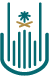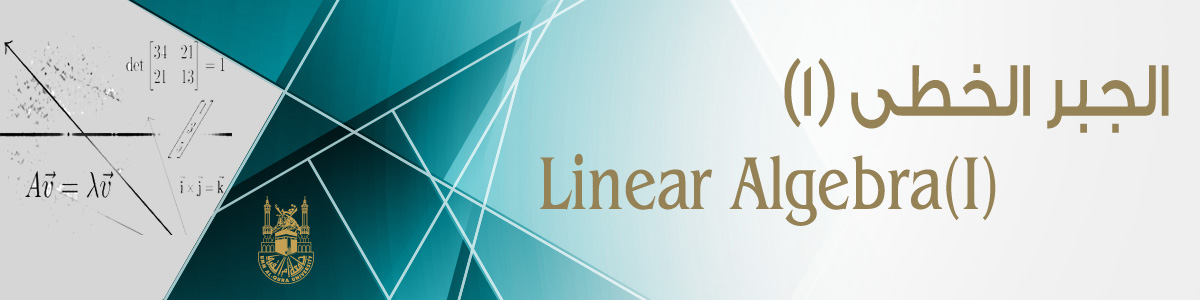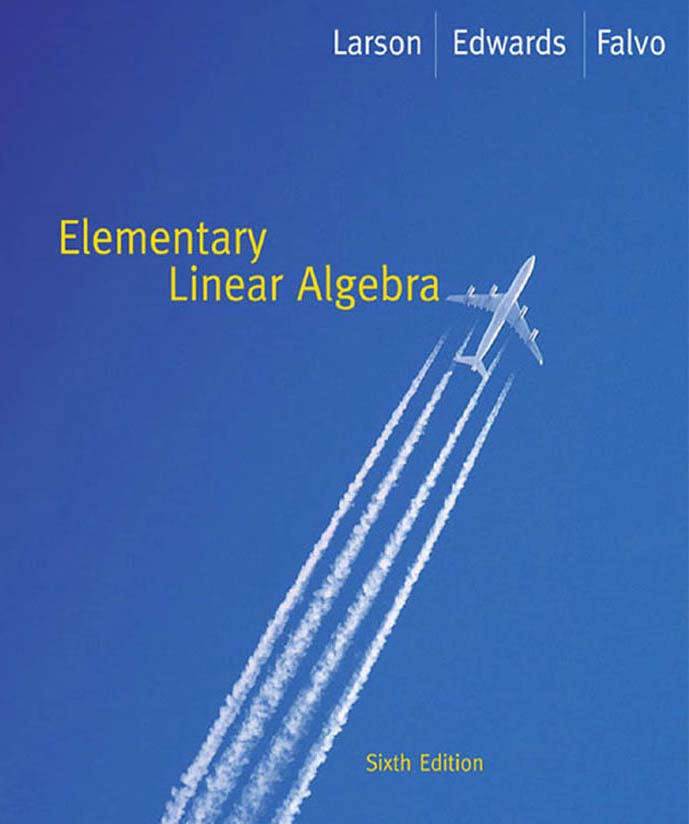# جامعة أم القرى# الجبر الخطى (1)

- 2018/09/07## 1- محتوى المقرر

List of Topics

No. of

Weeks

Linear equations in linear algebra: systems of linear equations, consistent and inconsistent systems of linear equations, examples

1-2

Elementary row operations, row reduction and echelon forms: examples

3-4

Matrix Algebra: Matrix operations,  Properties of matrix multiplication, the inverse of a matrix (invertible matrix theorem), elementary matrices, column space and null space of a matrix: examples

5-7

Determinants: Recursive definition of a determent, properties of determinants.  Applications:Cramer's rule and volume.

8-9

Vector spaces: Definition, examples, substructures, and linear transformations of vector spaces examples

10-11

Linearly independence and basis of a vector space: examples

12-13

Eigen values and Eigenvectors of matrices , Orthogonality and least Squares

14-15

## 2- الكتاب المقترح

### أ-الغلاف:### ب-المحتويات: (اضغط هنا لتحميل محتويات الكتاب).

جـ - التمارين المقررة.

جار التحميل